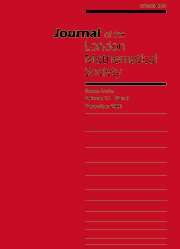HomeJournal of the London Mathematical Society

# the sum-of-digits function of squares

Published online by Cambridge University Press:  04 October 2005

## Abstract

the set of squares $n^2$, $n<2^k$, is considered and the sum of binary digits $s(n^2)$ is split up into two parts $s_{[<k]}(n^2)+s_{[\ge k]}(n^2)$, where $s_{[<k]}(n^2) = s(n^2{\rm mod}2^k)$ collects the first $k$ digits and $s_{[\ge k]}(n^2) = s(\lfloor n^2/2^k\rfloor)$ collects the remaining digits. very precise results on the distribution of $s_{[<k]}(n^2)$ and $s_{[\ge k]}(n^2)$ are presented. for example, asymptotic formulae are provided for the numbers $\#\{n< 2^k{:} s_{[<k]}(n^2) = m\}$ and $\#\{n< 2^k {:} s_{[\ge k]}(n^2) = m\}$ and it is shown that these partial sum-of-digits functions are asymptotically equidistributed in residue classes. these results are prompted by a conjecture by gelfond saying that the (total) sum-of-digits function $s(n^2)$ is asymptotically equidistributed in residue classes.

## Keywords

Type
notes and papers
Information
Journal of the London Mathematical Society , October 2005 , pp. 273 - 292
the london mathematical society 2005

## Access options

Get access to the full version of this content by using one of the access options below.

## Footnotes

this research was supported by the austrian science foundation fwf, grant s8302-mat.

### Full text views

Full text views reflects PDF downloads, PDFs sent to Google Drive, Dropbox and Kindle and HTML full text views.

Total number of HTML views: 0
Total number of PDF views: 2 *
View data table for this chart

* Views captured on Cambridge Core between September 2016 - 1st December 2020. This data will be updated every 24 hours.

Hostname: page-component-6d4bddd689-54zsc Total loading time: 0.361 Render date: 2020-12-01T15:50:40.151Z Query parameters: { "hasAccess": "0", "openAccess": "0", "isLogged": "0", "lang": "en" } Feature Flags last update: Tue Dec 01 2020 15:44:26 GMT+0000 (Coordinated Universal Time) Feature Flags: { "metrics": true, "metricsAbstractViews": false, "peerReview": true, "crossMark": true, "comments": true, "relatedCommentaries": true, "subject": true, "clr": false, "languageSwitch": true }

# Send article to Kindle

Note you can select to send to either the @free.kindle.com or @kindle.com variations. ‘@free.kindle.com’ emails are free but can only be sent to your device when it is connected to wi-fi. ‘@kindle.com’ emails can be delivered even when you are not connected to wi-fi, but note that service fees apply.

Find out more about the Kindle Personal Document Service.

the sum-of-digits function of squares
Available formats
×

# Send article to Dropbox

To send this article to your Dropbox account, please select one or more formats and confirm that you agree to abide by our usage policies. If this is the first time you use this feature, you will be asked to authorise Cambridge Core to connect with your <service> account. Find out more about sending content to Dropbox.

the sum-of-digits function of squares
Available formats
×

# Send article to Google Drive

To send this article to your Google Drive account, please select one or more formats and confirm that you agree to abide by our usage policies. If this is the first time you use this feature, you will be asked to authorise Cambridge Core to connect with your <service> account. Find out more about sending content to Google Drive.

the sum-of-digits function of squares
Available formats
×
×